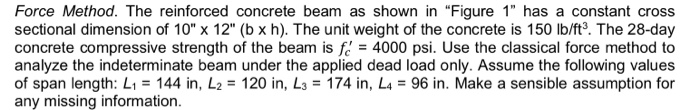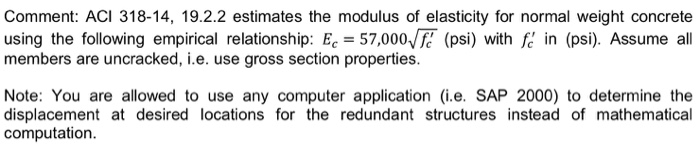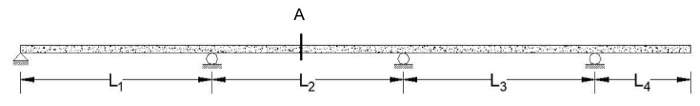# Please solve completely and correctly, I will give thumbs up! Force Method. The reinforced concrete beam as sh...

###### Question:

please solve completely and correctly, I will give thumbs up!Force Method. The reinforced concrete beam as shown in “Figure 1" has a constant cross sectional dimension of 10" x 12" (b x h). The unit weight of the concrete is 150 lb/ft3. The 28-day concrete compressive strength of the beam is = 4000 psi. Use the classical force method to analyze the indeterminate beam under the applied dead load only. Assume the following values of span length: L1 = 144 in, L2 = 120 in, L.-174 in, L4-96 in. Make a sensible assumption for any missing information
Comment: ACI 318-14, 19.2.2 estimates the modulus of elasticity for normal weight concrete using the following empirical relationship: Ec 57,000f (psi) with f in (psi). Assume all members are uncracked, i.e. use gross section properties. Note: You are allowed to use any computer application (i.e. SAP 2000) to determine the displacement at desired locations for the redundant structures instead of mathematical computation.
4 3

#### Similar Solved Questions

##### FORUM DESCRIPTION Complete your required discussion prompt Fungi tend to reproduce sexually when nutrients are limited...
FORUM DESCRIPTION Complete your required discussion prompt Fungi tend to reproduce sexually when nutrients are limited or other conditions are unfavorable, but they reproduce asexually in more ideal conditions. Why is this strategy successful? Grading Infermation...
##### Please answer with work Determine the limit at infinity. - 15x2 - 8x + 13 2)...
Please answer with work Determine the limit at infinity. - 15x2 - 8x + 13 2) lim X-00 -5x2 + + 3x + 2 2) A) 3 B) - c) 12 D) 1...
##### In 4. Suppose that {Xk, k > 1} is a sequence of i.i.d. random variables with...
in 4. Suppose that {Xk, k > 1} is a sequence of i.i.d. random variables with P(X1 = +1) = 1. Let Sn = 2h=1 Xk (i.e. Sn, n > 1 is a symmetric simple random walk with steps Xk, k > 1). (a) Compute E[S+1|X1, ... , Xn] for n > 1. Hint: Check out Example 3.8 in the lecture notes (Version Mar/...